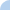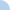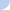# Regression lines and correlation r: session correlation and regression

Use the following: The least-squares regression line based on 43 observations of female white rats is y = 95 + 32x, where x is the weeks after birth and y is weight in grams. The correlation r is 0.78. Directions: Answer the following questions, and press the submit button when you are done. Use the following: The least-squares regression line based on 43 observations of female white rats is y = 95 + 32x, where x is the weeks after birth and y is weight in grams. The correlation r is 0.78.First name Last nameDavid Farnsworth

 This activity was created by a Quia Web subscriber. Learn more about QuiaCreate your own activities